# Frequency Compensation of Op-amp and why it is important in your Op-Amp Circuits

Published  September 27, 2019   0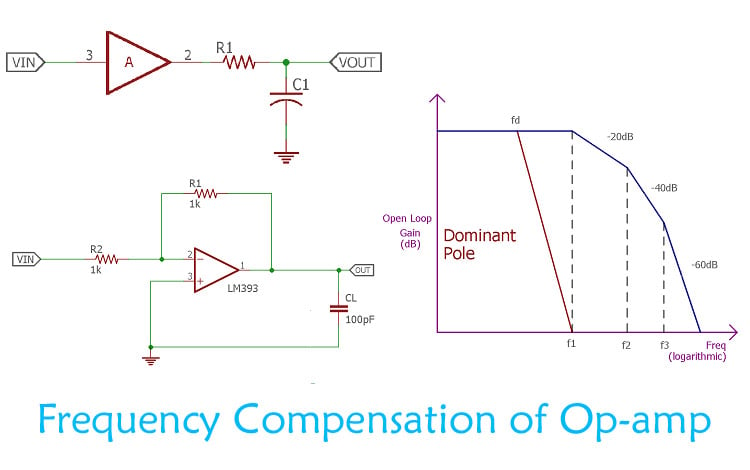Operational Amplifiers or Op-Amps are considered as the workhorse of Analog Electronic Designs. Back from the Analog computers era, Op-Amps have been used for mathematical operations with analog voltages hence the name operational amplifier. Till date Op-Amps are extensively used for voltage comparison, differentiation, integration, summation and many other things. Needless to say, the Operational Amplifier circuits are very easy to implement for different purposes but it has few limitations that often leads to complexity.

The major challenge is to improve the stability of an op-amp in a wide bandwidth of applications. The solution is to compensate the amplifier in terms of frequency response, by using a frequency compensation circuit across the operational amplifier. The stability of an amplifier is highly dependent on different parameters. In this article let’s understand the importance of Frequency Compensation and how to use it in your designs.

### Quick Basics on Op-Amp

Before going straight into the advance application of operational amplifiers and how to stabilize the amplifier using frequency compensation technique, let's explore a few basic things about the operational amplifier.

An amplifier can be configured as an open-loop configuration or a closed-loop configuration. In an open-loop configuration, there are no feedback circuits are associated with it. But in a closed-loop configuration, the amplifier needs feedback to work properly. The operational can have negative feedback or positive feedback. If the feedback network analog across the op-amp’s positive terminal, it is called positive feedback. Otherwise, negative feedback amplifiers have the feedback circuitry connected across negative terminal.

### Why do we need Frequency Compensation in Op-Amps?

Let's see the below amplifier circuit. It is a simple negative feedback non-inverting Op-Amp circuit. The circuit is connected as a unity-gain follower configuration.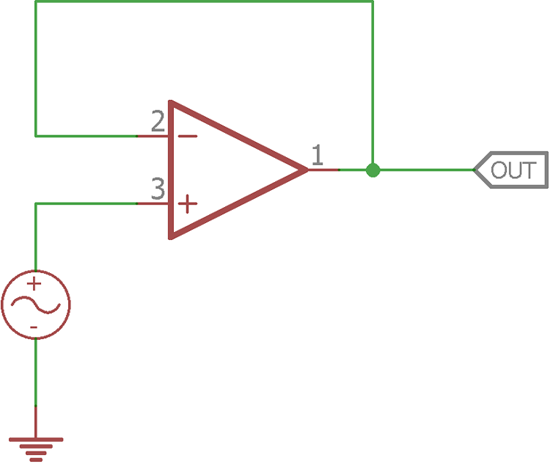The above circuit is very common in electronics. As we all know, amplifiers have very high input impedance across the input and could provide a reasonable amount of current across the output. Therefore, operational amplifiers can be driven using low signals to drive loads of higher current.

But what is the maximum current the op-amp could deliver to drive the load safely? The above circuit is good enough to drive pure resistive loads (ideal resistive load) but if we connect a capacitive load across the output, the op-amp will become unstable and based on the value of load capacitance at worst case the op-amp might even start to oscillate.

Let’s explore why the op-amp gets unstable when a capacitive load is connected across the output. The above circuit can be described as a simple formula -

`Acl= A / 1+Aß`

Acl is the closed-loop gain. A is the open-loop gain of the amplifier. Theis the feedback factor. That means how much the output is going back to the input of the amplifier. As the amplifier is unity gain amplifier, the feedback factor is 1 hence all of the output can be considered as going back to the input.

For the explanation of the Aß​, let's draw the negative feedback amplifier in a different point of view.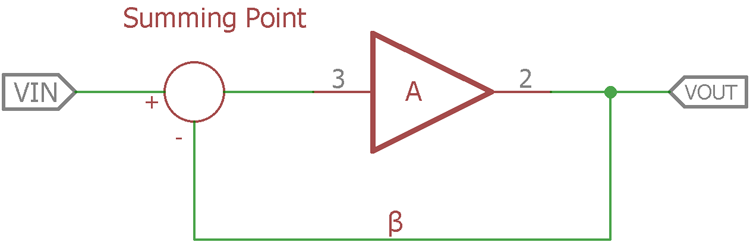The above image is a representation of the formula and negative feedback amplifier circuit. It is exactly identical with the traditional negative amplifier stated previously. They both share AC input on the positive terminal, and both have the same feedback in the negative terminal. The circle is the summing junction has two inputs, one from the input signal and the second one from the feedback circuit. Well, when the amplifier is working in negative feedback mode, the complete output voltage of the amplifier is flowing through the feedback line to the summing junction point. At the summing junction, feedback voltage and the input voltage is added together and feeded back into the input of the amplifier.

The image is divided into two gain stage. Firstly, it is showing complete closed-loop circuit as this is a closed-loop network and also the op-amps open-loop circuit because the op-amp showing A is a standalone open circuit, the feedback is not directly connected.

The output of the summing junction is further amplified by the op-amp open-loop gain. Therefore, if this complete thing is represented as a mathematical formation, the output across the summing junction is -

```Vin - VoutßNow the output of the amplifier will be -

Vout = open loop gain x (Vin - Voutß)
Or,
Vout = A(Vin - Voutß)
Vout = AVin - VoutAß
Vout + VoutAß= AVin
Vout (1+Aß) = AVin
Vout/Vin = A/(1+Aβ)```

### Instability Problem in Op-Amp

Therefore, the above circuit which is represented as the negative feedback amplifier has a transfer function of A/1+Aβ where Aß  is the loop gain factor. If at any situation, the 1+Aß becomes 0, the amplifier closed-loop gain or the transfer function will become infinity. But the closed-loop gain is limited as the power supply which is connected across the op-amp is limited hence the Amplifier will become unstable.

Now, for a negative feedback amplifier, the phase shift of the input and output is 180 degrees. When a capacitive load is connected across the amplifier, it can alter the phase by adding an additional pole across the op-amp output resulting in a negative to positive feedback conversion. The loop gain gets 1 at the 180-degree phase shift and induces instability.

Instability of the amplifier provides poor phase margin and hampered slew rate which results in an unnatural behavior across the op-amp output. The output oscillates and creates ringing effects when switching the output state. Since practically there are no ideal loads, resistive loads are not ideally resistive even perfectly made circuits have lots of capacitance as well as inductance. The outcome is poor phase response at high frequency and instability.

### How to deal with Op-Amp Instability?

The solution is not a straightforward way. To find out the problem or to check whether the amplifier is stable or not, one needs to find out the amplifier’s phase at unity loop gain. The Solution is to provide frequency compensation to the op-amp. This is a useful technique to overcome the instability of the op-amp as well as improve the step response of the circuit.

### Types of Op-Amp Frequency Compensation

There are different types of frequency compensation techniques used in electronics. However, all techniques are categorized into two basic types of compensation technique. The first one is external compensation across the op-amp and the second one is the internal compensation technique.

### External Frequency Compensation in Op Amp

External compensation techniques vary depending on the application, type of amplifier used and many other things. The easiest way is to use out-of loop compensation technique or in-loop compensation technique.

Out of the loop compensation technique uses a simple resistor to isolate the capacitive load with the op-amp, lowering the capacitive loading of the op-amp. The resistor typically varies from 10-50 Ohms but the increase in isolated resistor effects the op-amp bandwidth. The bandwidth of the op-amp drastically reduced to a very low value. One of the popular ways of out of the loop frequency compensation techniques is to use Dominant pole compensation technique.

1. Dominant pole Compensation

This technique uses a simple RC network connected across the output of the operational amplifier circuit. A sample dominant pole compensation circuit is shown below.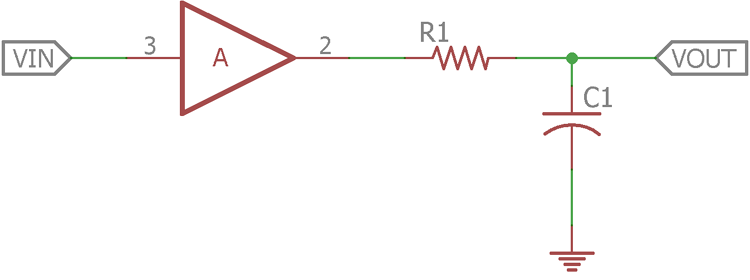This works great to overcome the instability issue. The RC network creates a pole at unity or 0dB gain that dominates or cancels out other high-frequency poles effect. The transfer function of the dominant pole configuration is –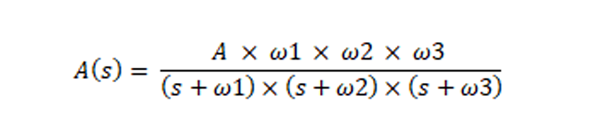Where, A(s)is the uncompensated transfer function, A is the open-loop gain, ώ1,ώ2, and ώ3 are the frequencies where the gain roll-off at -20dB, -40dB, -60dB respectively. The Bode plot below shows what happens if the dominant pole compensation technique is added across the op-amp output, where fd is the dominant pole frequency.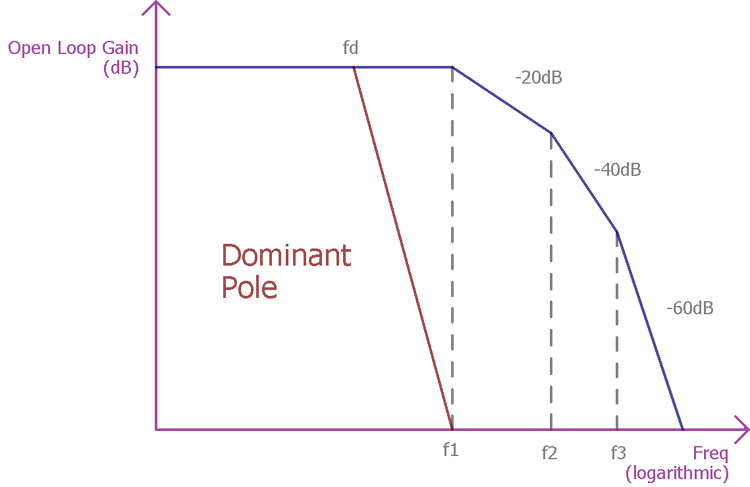2. Miller compensation

Another effective compensation technique is the miller compensation technique and it is an in-loop compensation technique where a simple capacitor is used with or without load isolation resistor (Nulling resistor). That means a capacitor is connected in the feedback loop to compensate the op-amp frequency response.

The miller compensation circuit is shown below. In this technique, a capacitor is connected to the feedback with a resistor across the output.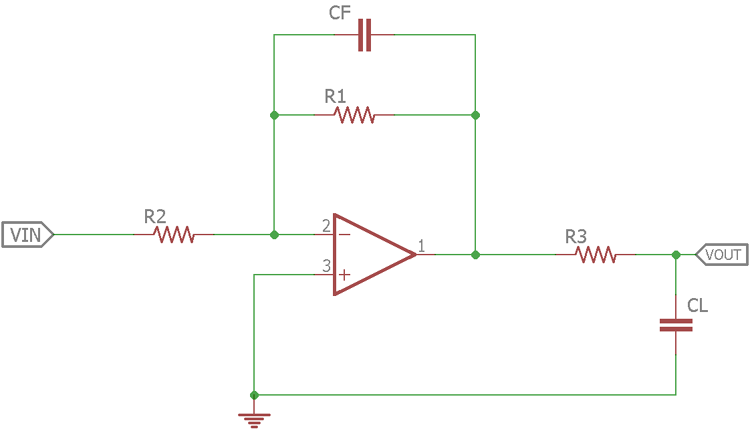The circuit is a simple negative feedback amplifier with inverting gain dependent on R1 and R2. The R3 is the null resistor and the CL is the capacitive load across the op-amp output. CF is the feedback capacitor which is used for the compensation purposes. The Capacitor and the resistor value depend on the type of amplifier stages, pole compensation, and the capacitive load.

### Internal Frequency Compensation Techniques

Modern operational amplifiers have internal compensation technique. In the internal compensation technique, a small feedback capacitor is connected inside of the op-amp IC between the second stages Common emitter transistor. For example, the below image is the internal diagram of popular op-amp LM358.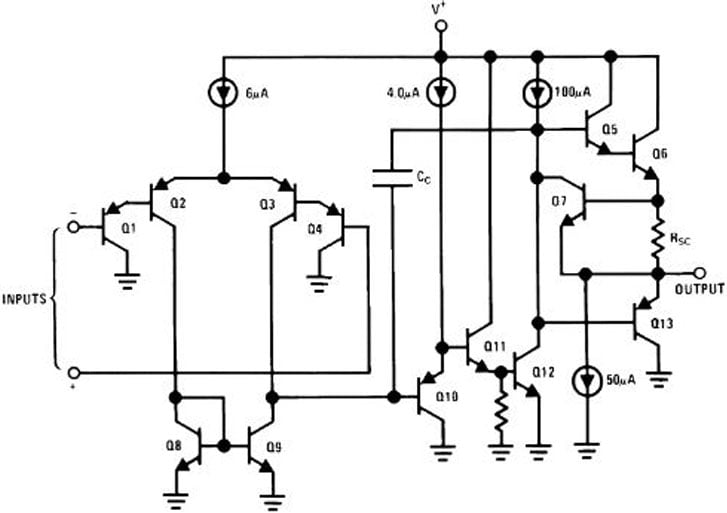The Cc capacitor is connected across the Q5 and Q10. It is the compensation Capacitor (Cc).  This compensation capacitor improves the stability of the amplifier and as well as prevent the oscillation and ringing effect across the output.

### Frequency Compensation of Op-amp – Practical simulation

To understand Frequency compensation more practically let’s try to simulate it by considering the below circuit –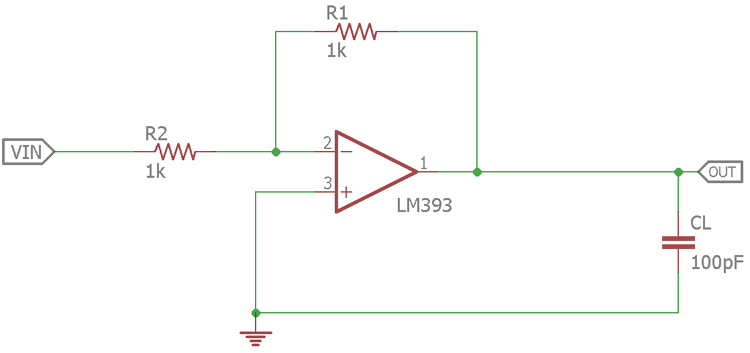The circuit is a simple negative feedback amplifier using LM393. This op-amp does not have any compensation capacitor inbuilt. We will simulate the circuit in Pspice with a 100pF of capacitive load and will check how it will perform in low and high-frequency operation.

To check this, one needs to analyze the open-loop gain and phase margin of the circuit. But it is a bit tricky for the pspice since simulating the exact circuit, as shown above, will represent its closed-loop gain. Therefore special considerations need to be taken. The step to converting the above circuit for open-loop gain simulation (gain vs phase) in pspice is stated below,

1. The input is grounded to obtain the feedback response; closed-loop input to output is ignored.
2. Inverting input is broken into two parts. One is the voltage divider and another one is the negative terminal of the op-amp.
3. Two parts are renamed to create two separate nodes and identification purposes during the simulation phase. Voltage divider section is renamed as feedback and the negative terminal is renamed as Inv-input. (Inverting input).
4. These two broken nodes are connected with a 0V DC voltage source. This is done because, from the term of DC voltage, both nodes have same voltage which is essential for the circuit to satisfy the current operating point requirement.
5. Adding the voltage source with a 1V of the AC stimulus. This forces the two individual nodes voltage difference to become 1 during the AC analysis. One thing is essential in this case, that the ratio of the feedback and the inverting input is dependable on the circuits open-loop gain.

After making the above steps, the circuit looks like this -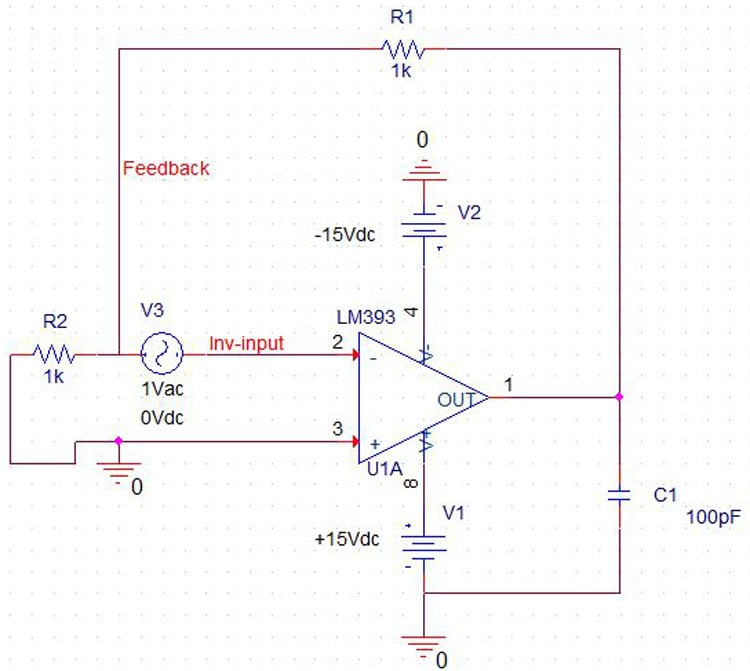The circuit is powered using 15V +/- power supply rail. Let's simulate the circuit and check its output bode plot.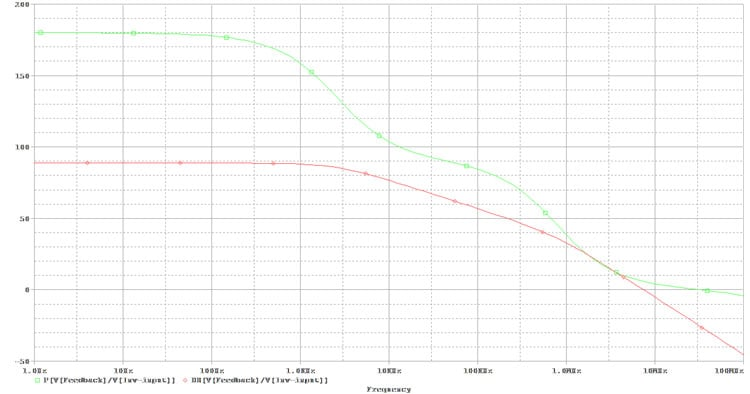Since the circuit has no frequency compensation, as expected the simulation is showing high gain at low frequency and low gain at high frequency. Also, it is showing very poor phase margin. Let's see what is the phase at 0dB gain.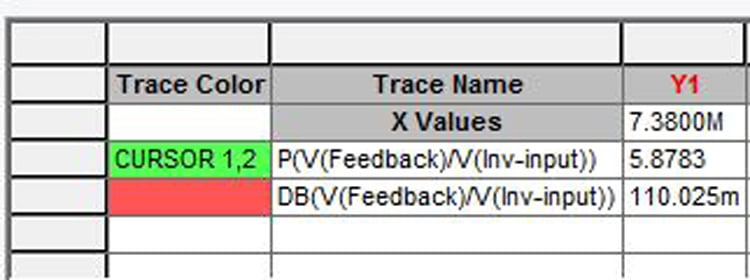As you can see even at 0dB gain or unity gain crossover, the op-amp is providing 6 degrees of phase shift at just only 100pF capacitive load.

Now let’s improvise the circuit by adding a frequency compensation resistor and capacitor to create miller compensation across the op-amp and analyze the result. A 50 Ohms of null resistor is placed across the op-amp and the output with a 100pF compensation capacitor.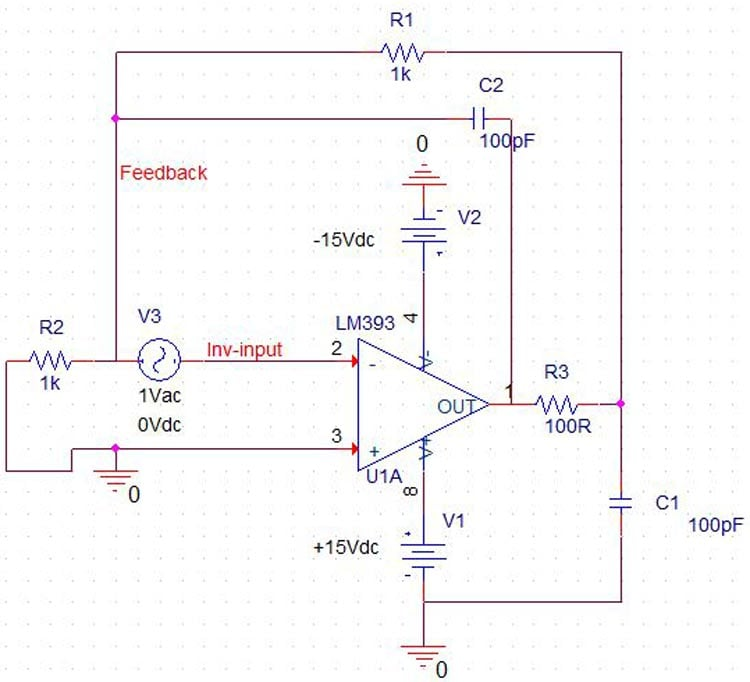The simulation is done and the curve looks like the below,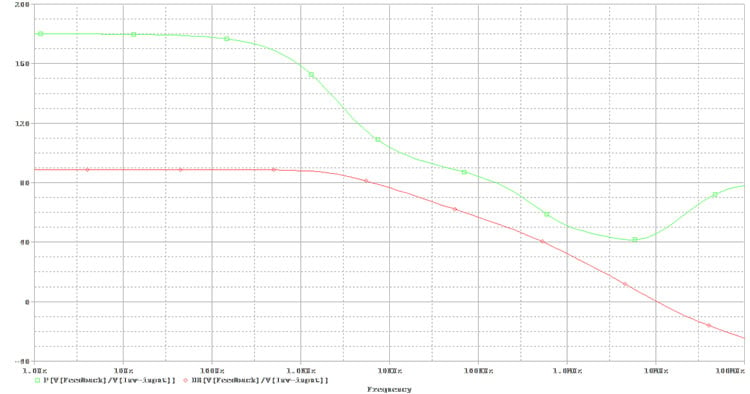The Phase curve is much better now. The phase shift at 0dB gain is almost 45.5 degrees. The amplifier stability is highly increased using the frequency compensation technique. Therefore, it is proven that the frequency compensation technique is highly recommended for the better stability of the op-map. But the Bandwidth will decrease.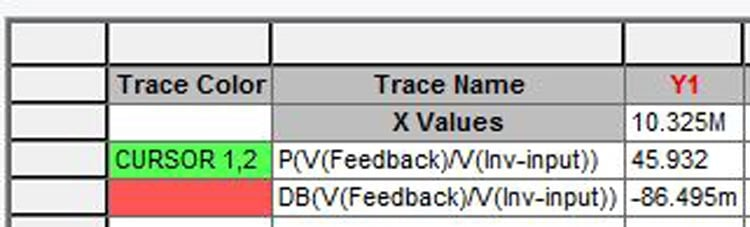Now we understand the importance of frequency compensation of opamp and how to use it in our Op-Amp designs to avoid instability problems. Hope you enjoyed reading the tutorial and learnt something useful. If you have any questions leave them in our forums or in the comment section below.